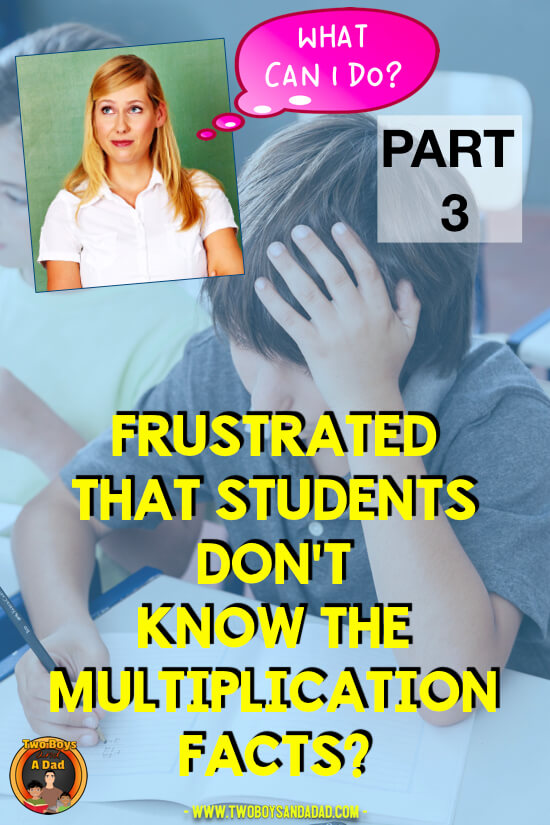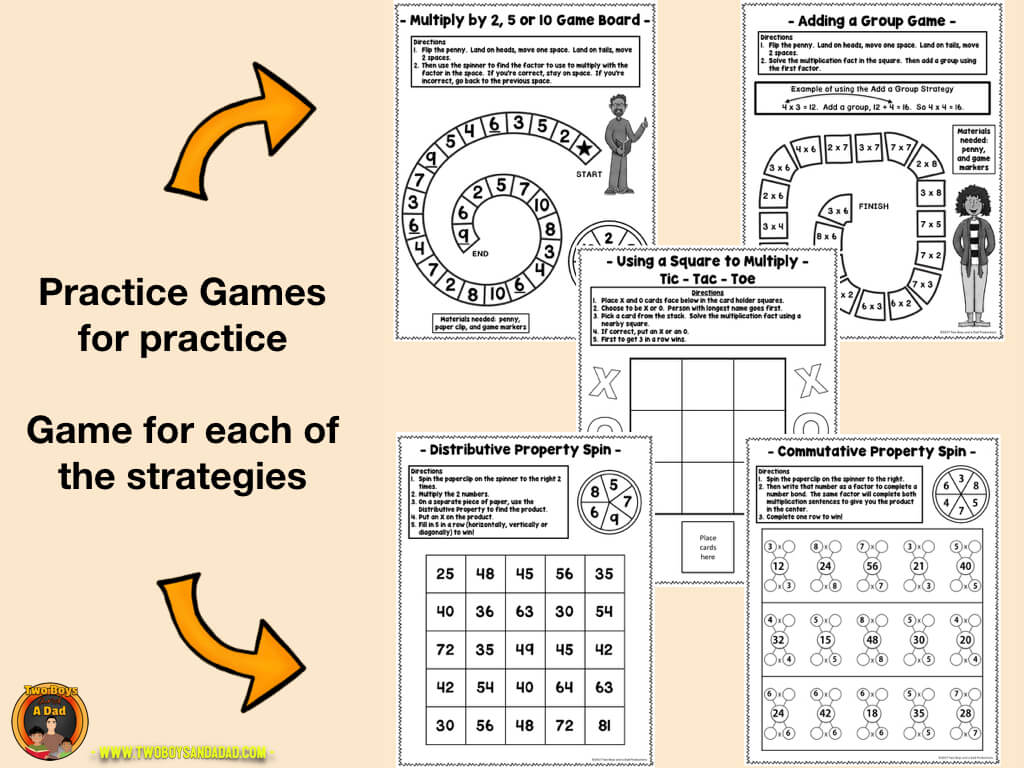# Frustrated That Students Don’t Know the Multiplication Facts? Part 3 The Derivative Strategies

In the final part of this three-part series on multiplication fluency, let’s explore the Derivative Strategies.  These are the strategies that are taught AFTER the student has demonstrated proficiency in the Foundation Strategies (see the previous post for an explanation of the Foundation Strategies).

The Derivative Strategies are the final steps that will eventually lead to multiplication fluency as well as, a better conceptual understanding of multiplication.## THE DERIVATIVE STRATEGIES:  Halving and Doubling for Multiplication Fluency

These are the strategies that continue to build that conceptual understanding of multiplication while building fluency over time.  The first strategy to teach is the halving and doubling strategy.  Why?

This strategy works with even factors.  So, if a student has learned and has fluency with the facts for the two times table, then he or she is ready to use this strategy to learn the facts for the four multiplication table.

Watch this example of a student using the halving and doubling strategy.

As you can see, knowing the 2s helps with learning the 4s because two is half of four.  The same strategy can be applied to learning the eight times table (though other strategies can be used as well).

## THE DERIVATIVE STRATEGIES:  Adding a Group for Multiplication Fluency

This is the second strategy to teach students to use.  Once the 2s and 4s are learned by doubling and halving, students can move on to learning the three times table by learning to add a group.  If the student knows the 2s timetable, then teach the student to use this strategy to learn the 3s.

Watch this video of a student using the Add a Group Strategy.

## THE DERIVATIVE STRATEGIES:  The Distributive Property Strategy

Of all the Properties of Multiplication, this is one of the hardest to teach.  Conceptually, students need to understand decomposing a number, partial products, and writing equations.  Not an easy task. Obviously, students need to be taught explicitly the Distributive Property of Multiplication.   I use this resource to teach the Distributive Property every year.  But whatever resources you use, once students are familiar with it, they can then practice the strategy for multiplying by seven and eight.

Before teaching this strategy, students really should be proficient in the facts for five.  Why? It is easier to decompose a number when one of the factors is five.  Multiplying by five leads to products with zero and five, which when added to the other partial product is easier to do mentally.

Watch this video of a student using the Distributive Property of Multiplication.

## THE DERIVATIVE STRATEGIES:  Other Strategies

There are several other strategies to teach which include subtracting a group, using a nearby square and the patterns for the nine times table.There is a definite sequence of teaching the multiplication tables.  I’ve created a resource that has everything you need to teach these strategies over time.Click to see the full product preview!

The Multiplication Fluency Strategies Resources includes teaching posters, student templates, practice pages, and games. See below for examples of each!Click to see the entire resource!

I’ve also included sample lesson plans for the Foundation Strategies and Derivative Strategies.  It also includes the teaching sequence for the multiplication tables and well as, explanations for each of the strategies.Click to see the entire resource!

Check out my Facebook page as well, as I will be posting more information and teaching multiplication, fact fluency, and more common core math topics!

Want more ideas and tips for teaching multiplication?  Check out my Pinterest Board for Multiplication Strategies.

# Don’t Go Yet!

Are you new to the 1:1 classroom setting? Then you’ll want to read my Valuable Tips for the 1:1 Classroom.

Check out how I use Google Classroom to present at Back to School Night for Parents.

Or register for the newsletter to receive this FREE Guide to Achieving Multiplication Fluency. Get it now by signing up for my newsletter below!###### Follow:

I’m a single dad raising two boys, 14 and 11. I’m also a veteran teacher of over 30 years who has taught first, second and third grades. I’m also a trained literacy coach, served as a mentor teacher and have a Bilingual Specialist Credential. I’m currently serving as a District Math Coach.